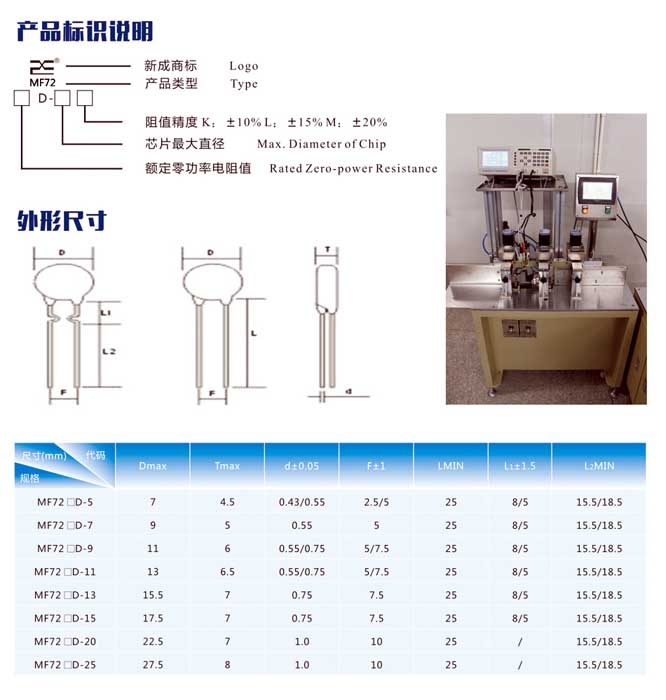China Thermal Protector Manufacturer

# MF72 Power Thermistor Supplier

Product Item: MF72 Power Thermistor Supplier
Category: Thermistor
Zero power resistance value RT (Ω)
RT refers to the resistance value measured at a specified temperature T using a measurement power that causes a change in resistance value to be negligible relative to the total measurement error.
The relationship between resistance value and temperature change is: RT = RN expB (1 / T-1 / TN)
RT: NTC thermistor resistance at temperature T (K)
RN: NTC thermistor resistance at rated temperature TN (K)
T: specified temperature (K)
B: Material constant of NTC thermistor, also known as thermal index
exp: exponent based on natural number e (e = 2.71828)

• PRODUCT DETAIL

Zero power resistance value RT (Ω)

RT refers to the resistance value measured at a specified temperature T using a measurement power that causes a change in resistance value to be negligible relative to the total measurement error.

The relationship between resistance value and temperature change is: RT = RN expB (1 / T-1 / TN)

RT: NTC thermistor resistance at temperature T (K)

RN: NTC thermistor resistance at rated temperature TN (K)

T: specified temperature (K)

B: Material constant of NTC thermistor, also known as thermal index

exp: exponent based on natural number e (e = 2.71828)

>>> Rated Zero Power Resistance R25 (Ω)

According to the national standard, the resistance value of the rated zero power resistor is the resistance value R25 measured by the NTC thermistor at a reference temperature of 25 ° C. This resistance value is the nominal resistance of the NTC thermistor. Generally, the NTC thermistor's resistance is also called the value.

>>> Material constant (thermal index) B value (K)

The B value is defined as:

B = [T1 * T2 / (T2-T1)] * ln (Rt1 / Rt2)

Rt1: Zero power resistance at temperature T1 (K)

Rt2: Zero power resistance at temperature T2 (K)

T1, T2: two specified temperatures (K)

Note: For commonly used NTC thermistors, the range of B value is generally between 2000K ~ 6000K.

The larger the B value, the smaller the residual resistance and the smaller the temperature rise during operation.The main technical parameters

 Spec Rated zero power resistance maximum steady-state current maximum residual resistance under steady-state current B25/85 B25/50 Thermal time constant Dissipation factor Operating temperature range Power Measurement rated power Resistance range  R25℃(Ω) Deviation (%) @25℃(A) R25℃(Ω) K Nominal value (K) Deviation (%) S mW/℃ ℃ mW mW 5D-5 5 1 0.5841 2700 < 18 > 6 -40~+150 10D-5 10 0.7 1.039 2700 < 18 > 6 -40~+150 15D-5 15 0.6 1.53 2800 < 18 > 6 -40~+150 20D-5 20 0.6 2.02 2800 < 18 > 6 -40~+150 22D-5 22 0.6 2.06 2800 < 18 > 6 -40~+150 30D-5 30 0.5 2.227 2800 < 18 > 6 -40~+150 33D-5 33 0.5 2.436 2800 < 18 > 6 -40~+150 50D-5 50 0.4 2.653 3000 < 18 > 6 -40~+150 60D-5 60 0.3 2.753 3000 < 18 > 6 -40~+150
Note: Not listed models can be customized
﻿
﻿